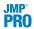• Select Analyze > Multivariate Methods > Partial Least Squares.
 •Select Analyze > Fit Model and select Partial Least Squares from the Personality menu. This approach enables you to do the following:
 – Enter categorical variables as Ys or Xs. Conduct PLS-DA by entering categorical Ys.
 – Add interaction and polynomial terms to your model.
 – Use the Standardize X option to construct higher-order terms using centered and scaled columns.
 – Save your model specification script.
 • Weight, Nest, Attributes, Transform, and No Intercept.
 • The following Macros: Mixture Response Surface, Scheffé Cubic, and Radial.
JMP Pro Partial Least Squares Launch Window (Imputation Method EM Selected)
 – If the validation column has two levels, the smaller value defines the training set and the larger value defines the validation set.
 – If the validation column has three levels, the values define the training, validation, and test sets in order of increasing size.
 –
If Impute Missing Data is not selected, rows that are missing observations on any X variable are excluded from the analysis and no predictions are computed for these rows. Rows with no missing observations on X variables but with missing observations on Y variables are also excluded from the analysis, but predictions are computed.
(Appears only when Impute Missing Data is selected) Select from the following imputation methods:
 – Mean: For each model effect or response column, replaces the missing value with the mean of the nonmissing values.
 – EM: Uses an iterative Expectation-Maximization (EM) approach to impute missing values. On the first iteration, the specified model is fit to the data with missing values for an effect or response replaced by their means. Predicted values from the model for Y and the model for X are used to impute the missing values. For subsequent iterations, the missing values are replaced by their predicted values, given the conditional distribution using the current estimates.

Help created on 9/19/2017